# Equation for circle points in 3D

• I
Nikkki
TL;DR Summary
Calculate the coordinates of consecutive points based on ABC points lying on a circle in 3D space
Hello,

I am trying to solve a problem and I would like to ask for help.

I have 3 points (A, B, C) in 3D space that are assumed to be on a circle.

EXAMPLE 1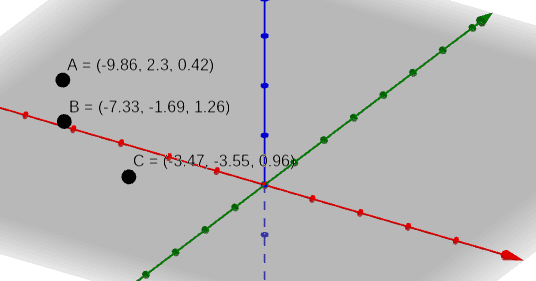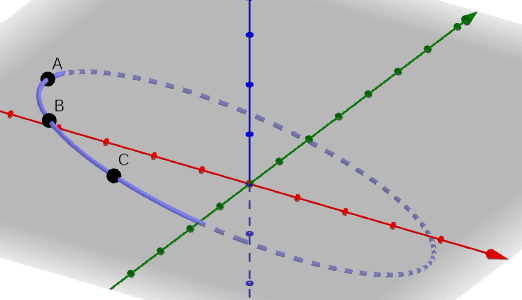EXAMPLE 2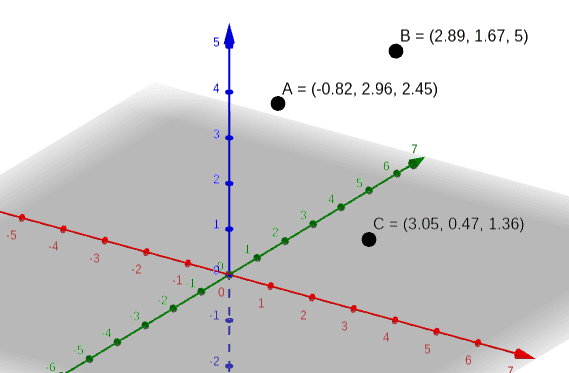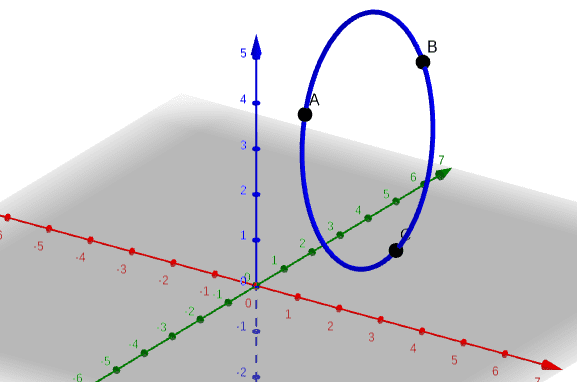My goal is to create an algebraic formula to calculate the coordinates for 10 points on a circle composed of ABC points at any distance from each other.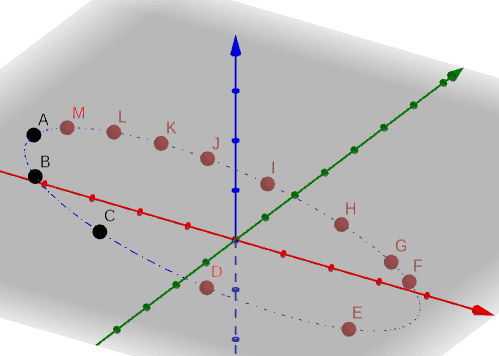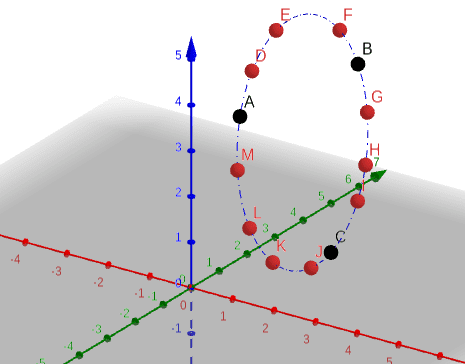MY IDEA

My first idea was to create a triangle inscribed in a circle from the ABC points and then the radius of the circle.
First, I calculate the lengths of the triangle's legs by recalculating the lengths of |AB| |AC| and |CB| vectors.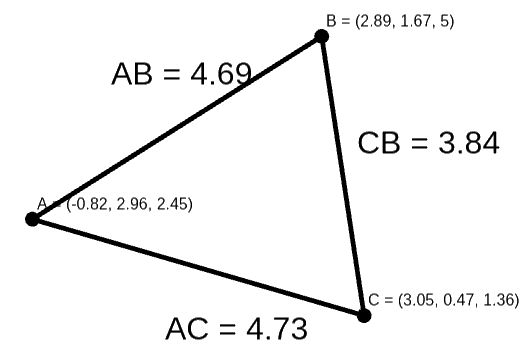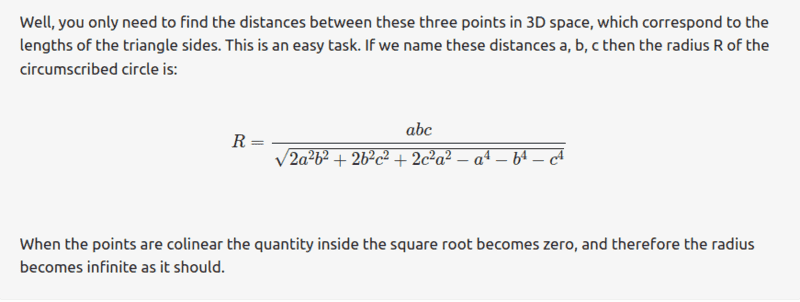And at this step, I have now stopped

Mentor
The center has a distance r to all these points. Can you find its coordinates as function of e.g. a, (b-a) and (c-a), e.g. the position of a and two sides? This is completely analogous to the two-dimensional problem.

•jim mcnamara
Nikkki
The center has a distance r to all these points. Can you find its coordinates as function of e.g. a, (b-a) and (c-a), e.g. the position of a and two sides? This is completely analogous to the two-dimensional problem.